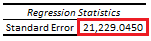# Linear Regression: Residual Standard Error

Last Update: February 21, 2022

Linear Regression: Residual Standard Error is used to evaluate linear regression goodness of fit by estimating its residual standard deviation.

As example, we can fit a three-variable multiple linear regression with formula . Then, we can estimate its residual standard error with formula . Residual mean squared error  with formula is estimated as residual sum of squares divided by residual degrees of freedom . Residual sum of squares  with formula  is estimated as the sum of squared regression residuals . Regression residuals  with formula  are estimated as differences between actual  and fitted  values. Residual degrees of freedom  with formula  are the number of observations  minus number of independent variables  minus constant term.

Below, we find an example of estimated residual standard error from multiple linear regression of house price explained by its lot size and number of bedrooms .Table 1. Microsoft Excel® estimated residual standard error from multiple linear regression of house price explained by its lot size and number of bedrooms.

Courses

My online courses are hosted at Teachable website.

For more details on this concept, you can view my Linear Regression Courses.

 Data Description: Sales prices of houses sold in the city of Windsor, Canada, during July, August and September, 1987.

Original Source: Anglin, P., and Gencay, R. (1996). Semiparametric Estimation of a Hedonic Price Function. Journal of Applied Econometrics, 11, 633–648.

Source: AER R Package HousePrices Object. Christian Kleiber and Achim Zeileis. (2008). Applied Econometrics with R. Springer-Verlag, New York.

+